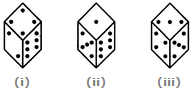# Non Verbal Reasoning - Cubes and Dice - Discussion

### Discussion :: Cubes and Dice - Section 2 (Q.No.2)

2.

Three different positions of a dice are shown below. How many dots lie opposite 2 dots?[A]. 1 [B]. 3 [C]. 5 [D]. 6

Explanation:

From figures (ii) and (iii), we conclude that 1, 6, 3 and 4 dots lie adjacent to 5 dots. Therefore, 2 dots must lie opposite 5 dots. Conversely, 5 dots must lie opposite 2 dots.

 Gobind Charan Banra said: (Apr 30, 2012) Sir, i think your annwer is wrong, in figure (1) shown that 2 and 6 is not opposite to 4. in figure (iii) shown that 5 and 3 in not opposite to 4.so i think if 2,3,5 and 6 is not opposite to 4. It is 1 which is opposite to 4. so i think answer should be [A] not [c].

 Raw said: (Oct 15, 2015) Only first two dice are needed to solve this. And the correct answer is indeed "C".